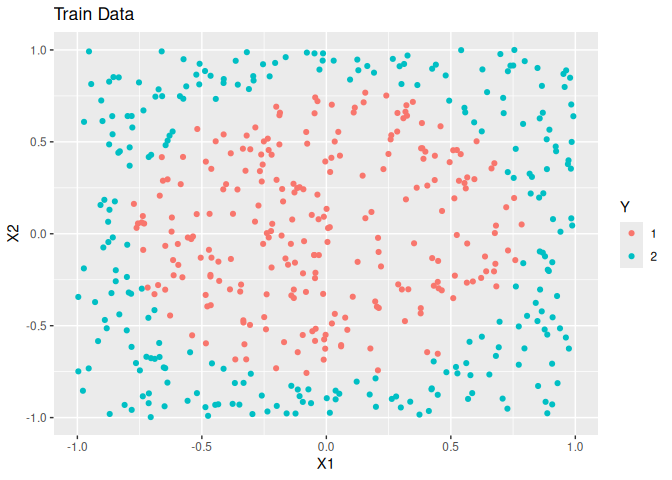# DANN

An Implementation of Hastie and Tibshirani’s Discriminant Adaptive Nearest Neighbor Classification in R.

## Installation

You can install the released version of dann from CRAN with:

``install.packages("dann")``

And the development version from GitHub with:

``````# install.packages("devtools")
devtools::install_github("gmcmacran/dann")``````

## Package Introduction

In k nearest neighbors, the shape of the neighborhood is always circular. Discriminant Adaptive Nearest Neighbor (dann) is a variation of k nearest neighbors where the shape of the neighborhood is data driven. The neighborhood is elongated along class boundaries and shrunk in the orthogonal direction to class boundaries. See Discriminate Adaptive Nearest Neighbor Classification by Hastie and Tibshirani. This package implements DANN and sub-DANN in section 4.1 of the publication and is based on Christopher Jenness’s python implementation.

## Example: Circle Problem

In this example, simulated data is made. The overall trend is a circle inside a square.

``````library(dann)
library(dplyr, warn.conflicts = FALSE)
library(ggplot2)
library(mlbench)

set.seed(1)

#Create training data
train <- mlbench.circle(500, 2) %>%
tibble::as_tibble()
colnames(train) <- c("X1", "X2", "Y")

ggplot(train, aes(x = X1, y = X2, colour = Y)) +
geom_point() +
labs(title = "Train Data")````````````
#Create test data
test <- mlbench.circle(500, 2) %>%
tibble::as_tibble()
colnames(test) <- c("X1", "X2", "Y")

ggplot(test, aes(x = X1, y = X2, colour = Y)) +
geom_point() +
labs(title = "Test Data")``````Next, a bit of data shaping is needed. dann is more matrix driven as opposed to dataframe driven. The predictor variables need to be one matrix. The observed labels need to be in another.

``````xTrain <- train %>%
select(X1, X2) %>%
as.matrix()

yTrain <- train %>%
pull(Y) %>%
as.numeric() %>%
as.vector()

xTest <- test %>%
select(X1, X2) %>%
as.matrix()

yTest <- test %>%
pull(Y) %>%
as.numeric() %>%
as.vector()``````

To train a model, the matrices and a few parameters are passed into dann. The argument neighborhood_size is the number of data points used to estimate a good shape of the neighborhood. The argument k is the number of data points used in the final classification. Overall, dann is a highly accurate model for this data set.

``````dannPreds <- dann(xTrain = xTrain, yTrain = yTrain, xTest = xTest,
k = 7, neighborhood_size = 50)
mean(dannPreds == yTest)
#>  0.964``````